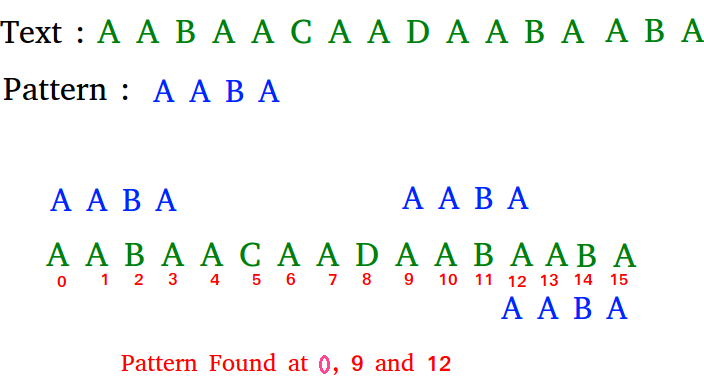Related Articles

# Naive algorithm for Pattern Searching

• Difficulty Level : Easy
• Last Updated : 14 Apr, 2021

Given a text txt[0..n-1] and a pattern pat[0..m-1], write a function search(char pat[], char txt[]) that prints all occurrences of pat[] in txt[]. You may assume that n > m
Examples:

```Input:  txt[] = "THIS IS A TEST TEXT"
pat[] = "TEST"
Output: Pattern found at index 10

pat[] =  "AABA"
Output: Pattern found at index 0
Pattern found at index 9
Pattern found at index 12```` `

Pattern searching is an important problem in computer science. When we do search for a string in notepad/word file or browser or database, pattern searching algorithms are used to show the search results.

Naive Pattern Searching:
Slide the pattern over text one by one and check for a match. If a match is found, then slides by 1 again to check for subsequent matches.

## C

 `// C program for Naive Pattern Searching algorithm``#include ``#include ` `void` `search(``char``* pat, ``char``* txt)``{``    ``int` `M = ``strlen``(pat);``    ``int` `N = ``strlen``(txt);` `    ``/* A loop to slide pat[] one by one */``    ``for` `(``int` `i = 0; i <= N - M; i++) {``        ``int` `j;` `        ``/* For current index i, check for pattern match */``        ``for` `(j = 0; j < M; j++)``            ``if` `(txt[i + j] != pat[j])``                ``break``;` `        ``if` `(j == M) ``// if pat[0...M-1] = txt[i, i+1, ...i+M-1]``            ``printf``(``"Pattern found at index %d \n"``, i);``    ``}``}` `/* Driver program to test above function */``int` `main()``{``    ``char` `txt[] = ``"AABAACAADAABAAABAA"``;``    ``char` `pat[] = ``"AABA"``;``    ``search(pat, txt);``    ``return` `0;``}`

## C++

 `// C++ program for Naive Pattern``// Searching algorithm``#include ``using` `namespace` `std;` `void` `search(``char``* pat, ``char``* txt)``{``    ``int` `M = ``strlen``(pat);``    ``int` `N = ``strlen``(txt);` `    ``/* A loop to slide pat[] one by one */``    ``for` `(``int` `i = 0; i <= N - M; i++) {``        ``int` `j;` `        ``/* For current index i, check for pattern match */``        ``for` `(j = 0; j < M; j++)``            ``if` `(txt[i + j] != pat[j])``                ``break``;` `        ``if` `(j == M) ``// if pat[0...M-1] = txt[i, i+1, ...i+M-1]``            ``cout << ``"Pattern found at index "``                 ``<< i << endl;``    ``}``}` `// Driver Code``int` `main()``{``    ``char` `txt[] = ``"AABAACAADAABAAABAA"``;``    ``char` `pat[] = ``"AABA"``;``    ``search(pat, txt);``    ``return` `0;``}` `// This code is contributed``// by Akanksha Rai`

## Java

 `// Java program for Naive Pattern Searching``public` `class` `NaiveSearch {` `    ``public` `static` `void` `search(String txt, String pat)``    ``{``        ``int` `M = pat.length();``        ``int` `N = txt.length();` `        ``/* A loop to slide pat one by one */``        ``for` `(``int` `i = ``0``; i <= N - M; i++) {` `            ``int` `j;` `            ``/* For current index i, check for pattern``              ``match */``            ``for` `(j = ``0``; j < M; j++)``                ``if` `(txt.charAt(i + j) != pat.charAt(j))``                    ``break``;` `            ``if` `(j == M) ``// if pat[0...M-1] = txt[i, i+1, ...i+M-1]``                ``System.out.println(``"Pattern found at index "` `+ i);``        ``}``    ``}` `    ``public` `static` `void` `main(String[] args)``    ``{``        ``String txt = ``"AABAACAADAABAAABAA"``;``        ``String pat = ``"AABA"``;``        ``search(txt, pat);``    ``}``}``// This code is contributed by Harikishore`

## Python3

 `# Python3 program for Naive Pattern``# Searching algorithm``def` `search(pat, txt):``    ``M ``=` `len``(pat)``    ``N ``=` `len``(txt)` `    ``# A loop to slide pat[] one by one */``    ``for` `i ``in` `range``(N ``-` `M ``+` `1``):``        ``j ``=` `0``        ` `        ``# For current index i, check``        ``# for pattern match */``        ``while``(j < M):``            ``if` `(txt[i ``+` `j] !``=` `pat[j]):``                ``break``            ``j ``+``=` `1` `        ``if` `(j ``=``=` `M):``            ``print``(``"Pattern found at index "``, i)` `# Driver Code``if` `__name__ ``=``=` `'__main__'``:``    ``txt ``=` `"AABAACAADAABAAABAA"``    ``pat ``=` `"AABA"``    ``search(pat, txt)` `# This code is contributed``# by PrinciRaj1992`

## C#

 `// C# program for Naive Pattern Searching``using` `System;` `class` `GFG {` `    ``public` `static` `void` `search(String txt, String pat)``    ``{``        ``int` `M = pat.Length;``        ``int` `N = txt.Length;` `        ``/* A loop to slide pat one by one */``        ``for` `(``int` `i = 0; i <= N - M; i++) {``            ``int` `j;` `            ``/* For current index i, check for pattern``            ``match */``            ``for` `(j = 0; j < M; j++)``                ``if` `(txt[i + j] != pat[j])``                    ``break``;` `            ``// if pat[0...M-1] = txt[i, i+1, ...i+M-1]``            ``if` `(j == M)``                ``Console.WriteLine(``"Pattern found at index "` `+ i);``        ``}``    ``}` `    ``// Driver code``    ``public` `static` `void` `Main()``    ``{``        ``String txt = ``"AABAACAADAABAAABAA"``;``        ``String pat = ``"AABA"``;``        ``search(txt, pat);``    ``}``}``// This code is Contributed by Sam007`

## PHP

 ``

## Javascript

 ``

Output:

```Pattern found at index 0
Pattern found at index 9
Pattern found at index 13 ```

What is the best case?
The best case occurs when the first character of the pattern is not present in text at all.

## C

 `txt[] = ``"AABCCAADDEE"``;``pat[] = ``"FAA"``;`

The number of comparisons in best case is O(n).
What is the worst case ?
The worst case of Naive Pattern Searching occurs in following scenarios.
1) When all characters of the text and pattern are same.

## C

 `txt[] = ``"AAAAAAAAAAAAAAAAAA"``;``pat[] = ``"AAAAA"``;`

2) Worst case also occurs when only the last character is different.

## C

 `txt[] = ``"AAAAAAAAAAAAAAAAAB"``;``pat[] = ``"AAAAB"``;`

The number of comparisons in the worst case is O(m*(n-m+1)). Although strings which have repeated characters are not likely to appear in English text, they may well occur in other applications (for example, in binary texts). The KMP matching algorithm improves the worst case to O(n). We will be covering KMP in the next post. Also, we will be writing more posts to cover all pattern searching algorithms and data structures.

?list=PLqM7alHXFySEQDk2MDfbwEdjd2svVJH9p